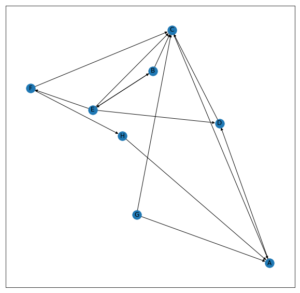Related Articles

# Hyperlink Induced Topic Search (HITS) Algorithm using Networxx Module | Python

• Last Updated : 22 Feb, 2021

Hyperlink Induced Topic Search (HITS) Algorithm is a Link Analysis Algorithm that rates webpages, developed by Jon Kleinberg. This algorithm is used to the web link-structures to discover and rank the webpages relevant for a particular search.
HITS uses hubs and authorities to define a recursive relationship between webpages. Before understanding the HITS Algorithm, we first need to know about Hubs and Authorities.

• Given a query to a Search Engine, the set of highly relevant web pages are called Roots. They are potential Authorities.
• Pages that are not very relevant but point to pages in the Root are called Hubs. Thus, an Authority is a page that many hubs link to whereas a Hub is a page that links to many authorities.

Algorithm –

-> Let number of iterations be k
-> Each node is assigned a Hub score = 1 and an Authority score = 1.
-> Repeat k times:

• Hub update : Each node’s Hub score =(Authority score of each node it points to).
• Authority update : Each node’s Authority score =(Hub score of each node pointing to it).
• Normalize the scores by dividing each Hub score by square root of the sum of the squares of all Hub scores, and dividing each Authority score by square root of the sum of the squares of all Authority scores. (optional)

Now, let’s see how to implement this algorithm using Networxx Module.
Let us consider the following Graph:On running HITS Algorithm with(without Normalization),

```Initially,
Hub Scores:        Authority Scores:
A -> 1             A -> 1
B -> 1             B -> 1
C -> 1             C -> 1
D -> 1             D -> 1
E -> 1             E -> 1
F -> 1             F -> 1
G -> 1             G -> 1
H -> 1             H -> 1

After 1st iteration,
Hub Scores:        Authority Scores:
A -> 1             A -> 3
B -> 2             B -> 2
C -> 1             C -> 4
D -> 2             D -> 2
E -> 4             E -> 1
F -> 1             F -> 1
G -> 2             G -> 0
H -> 1             H -> 1

After 2nd iteration,
Hub Scores:        Authority Scores:
A -> 2             A -> 4
B -> 5             B -> 6
C -> 3             C -> 7
D -> 6             D -> 5
E -> 9             E -> 2
F -> 1             F -> 4
G -> 7             G -> 0
H -> 3             H -> 1

After 3rd iteration,
Hub Scores:        Authority Scores:
A -> 5             A -> 13
B -> 9             B -> 15
C -> 4             C -> 27
D -> 13            D -> 11
E -> 22            E -> 5
F -> 1             F -> 9
G -> 11            G -> 0
H -> 4             H -> 3```

The Python package Networkx has an in-built function for running the HITS Algorithm. This would be visualized with reference to the above Graph.

## Python3

 `# importing modules``import` `networkx as nx``import` `matplotlib.pyplot as plt` `G ``=` `nx.DiGraph()` `G.add_edges_from([(``'A'``, ``'D'``), (``'B'``, ``'C'``), (``'B'``, ``'E'``), (``'C'``, ``'A'``),``                  ``(``'D'``, ``'C'``), (``'E'``, ``'D'``), (``'E'``, ``'B'``), (``'E'``, ``'F'``),``                  ``(``'E'``, ``'C'``), (``'F'``, ``'C'``), (``'F'``, ``'H'``), (``'G'``, ``'A'``),``                  ``(``'G'``, ``'C'``), (``'H'``, ``'A'``)])` `plt.figure(figsize ``=``(``10``, ``10``))``nx.draw_networkx(G, with_labels ``=` `True``)` `hubs, authorities ``=` `nx.hits(G, max_iter ``=` `50``, normalized ``=` `True``)``# The in-built hits function returns two dictionaries keyed by nodes``# containing hub scores and authority scores respectively.` `print``(``"Hub Scores: "``, hubs)``print``(``"Authority Scores: "``, authorities)`

Output:```Hub Scores:  {'A': 0.04642540386472174, 'D': 0.133660375232863,
'B': 0.15763599440595596, 'C': 0.037389132480584515,
'E': 0.2588144594158868, 'F': 0.15763599440595596,
'H': 0.037389132480584515, 'G': 0.17104950771344754}

Authority Scores:  {'A': 0.10864044085687284, 'D': 0.13489685393050574,
'B': 0.11437974045401585, 'C': 0.3883728005172019,
'E': 0.06966521189369385, 'F': 0.11437974045401585,
'H': 0.06966521189369385, 'G': 0.0}```

Attention geek! Strengthen your foundations with the Python Programming Foundation Course and learn the basics.

To begin with, your interview preparations Enhance your Data Structures concepts with the Python DS Course. And to begin with your Machine Learning Journey, join the Machine Learning – Basic Level Course

My Personal Notes arrow_drop_up